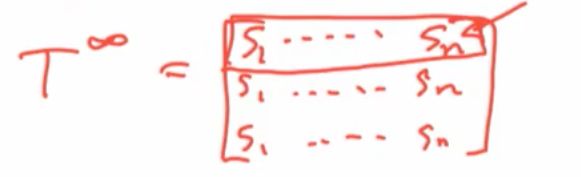# Theorem¶

Suppose T is strongly connected

T is convergent if and only if it is aperiodic

• "strongly connected" is going to say that from every given individual, there exists a directed path from i to j.

$\exists$ directed path i to j $\forall i, j$

T is convergent if and only if: lim $T^t = (1,1,...,1)^T s$ where s is the unique lhs(left hand side) eigenvector with eigenvalue 1# Proof¶

Defn: T is primitive if $T_{ij}^t > 0, \forall i,j$ after some t

• If T is strongly connected and stochastic then it is aperiodic if and only if it is primitive. (Perkins(1961))

• If T is strongly connected and primitive then lim $T^t = (1,1,...,1)^Ts$

where s is the unique lhs eigenvector with eigenvalue "1"(e.g. see Meyer(2000))

# Proof Cont'd¶

• So, strongly connected and aperiodic implies convergence.

• Converse comes from showing:

• If T is strongly connected, stochastic and convergent, then it is primitive

Let $S =$ lim $T^t$ by convergence

Then $ST =$ lim $T^t T = S$

• So each row is lhs eigenvector with eigenvalue "1": it is a positive vector by Perron-Frobenius theorem(an eigenvector of an irreducible non-negative matrix is strictly positive if and only if it is associated with its largest eigenvalue. This vector is unique if the matrix is primitive)

• So since S is all positive, T is primitive

• Since, T is primitive then Perron-Frobenius implies the eigenvector is unique, and all rows of S are the same s

# Aperiodicity Easy¶

• Aperiodicity is easy to satisfy:
• Have some agent weight him or herself
• Or have at least one communicating dyad and a transitive triple...